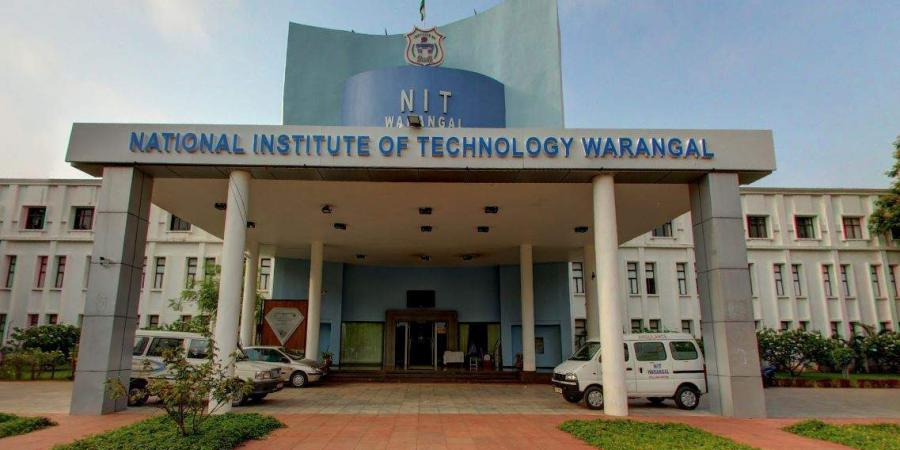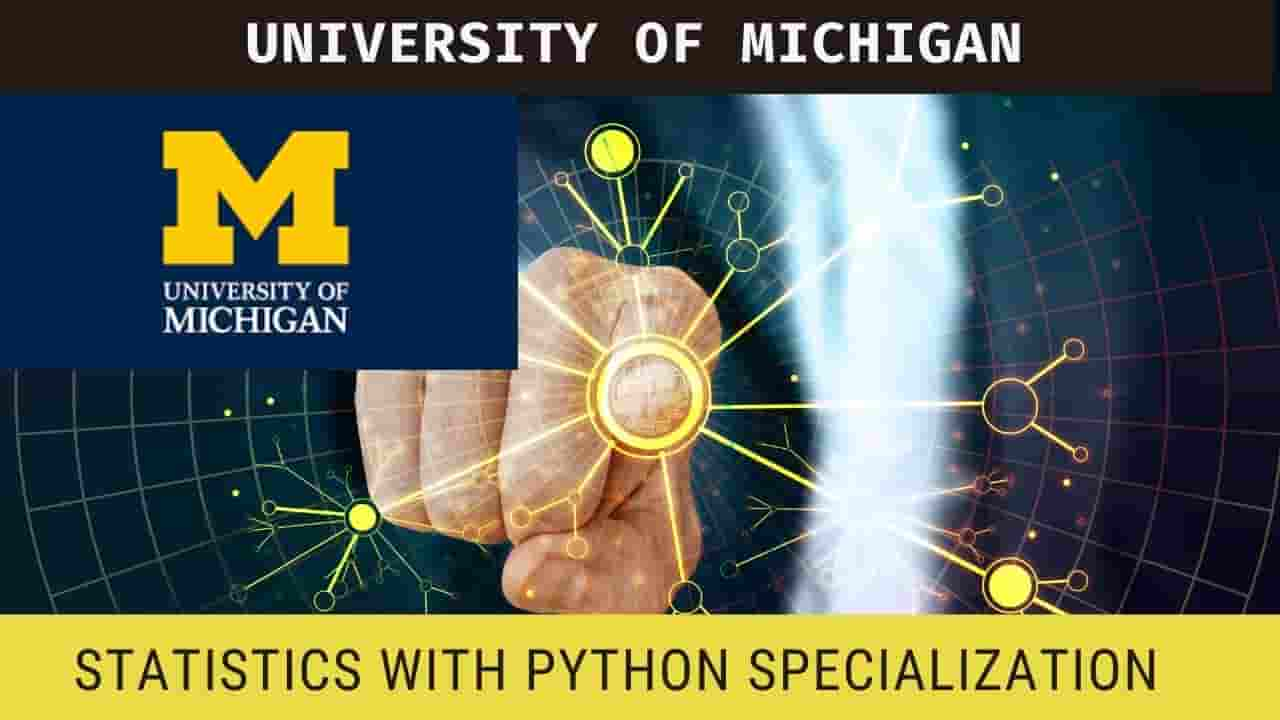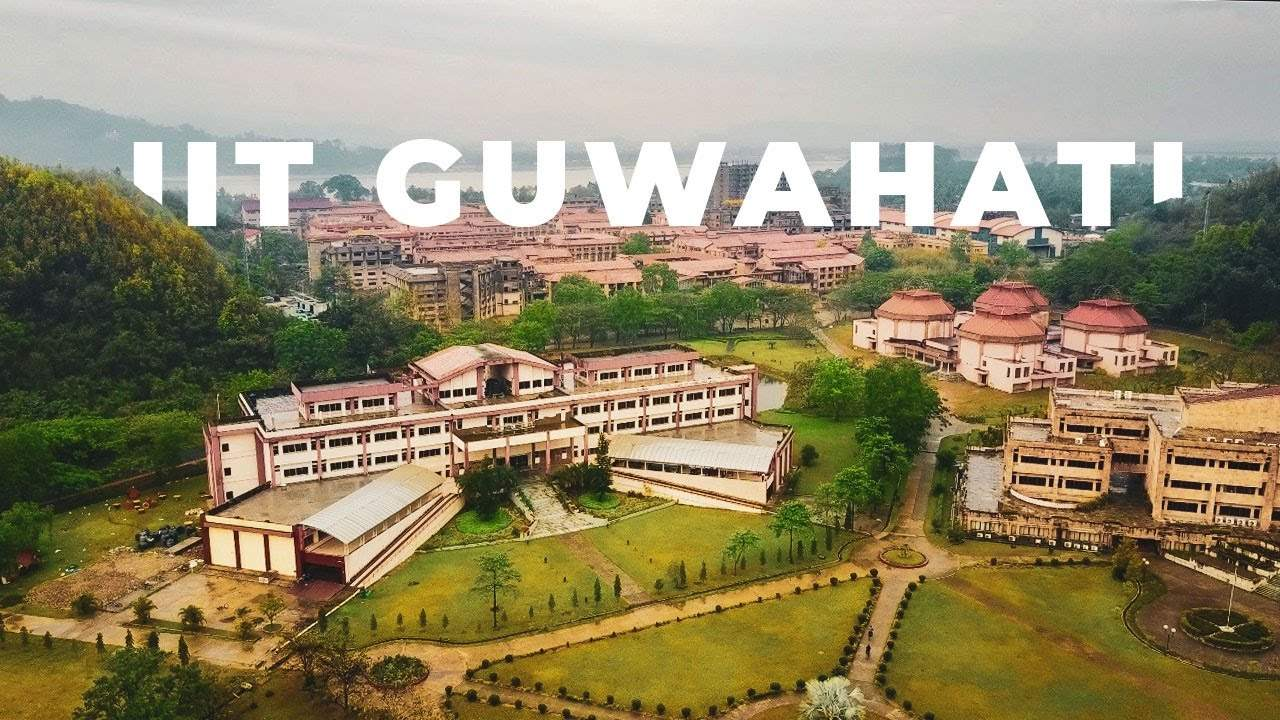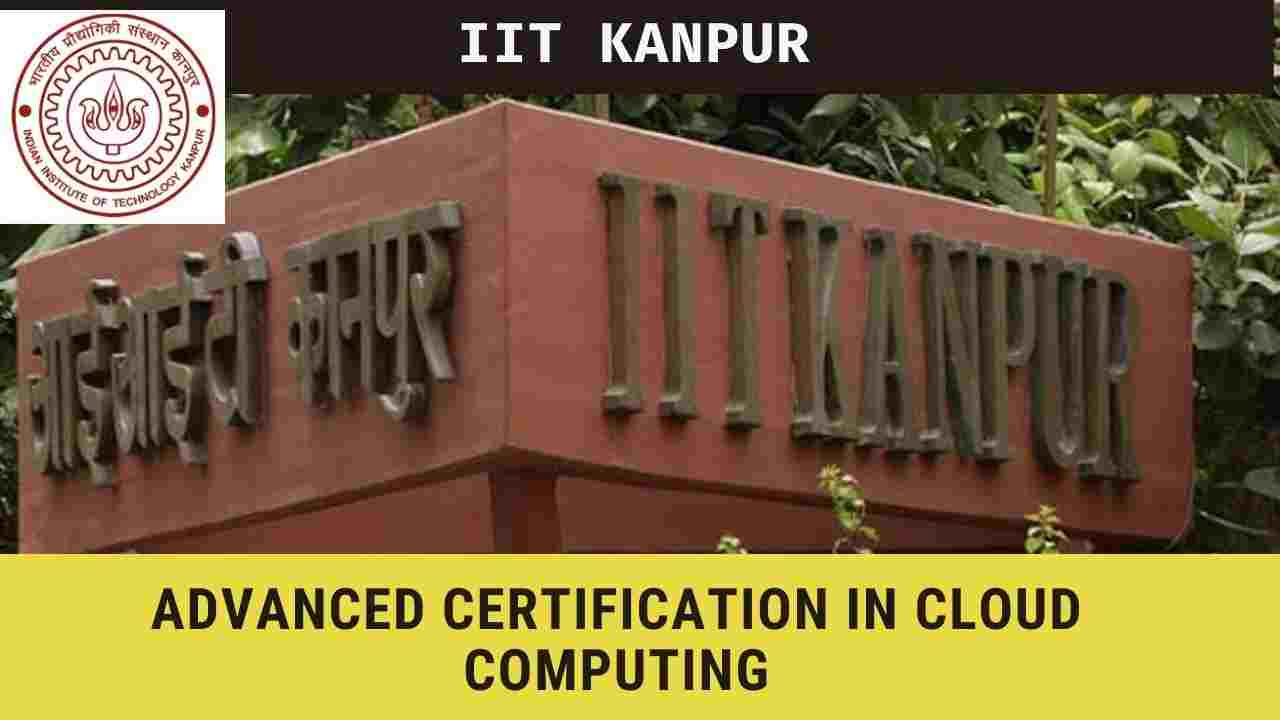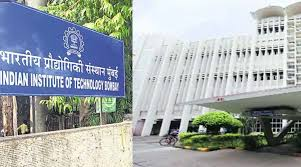# IIT Madras FREE course on the Data Science for Engineers by SWAYAM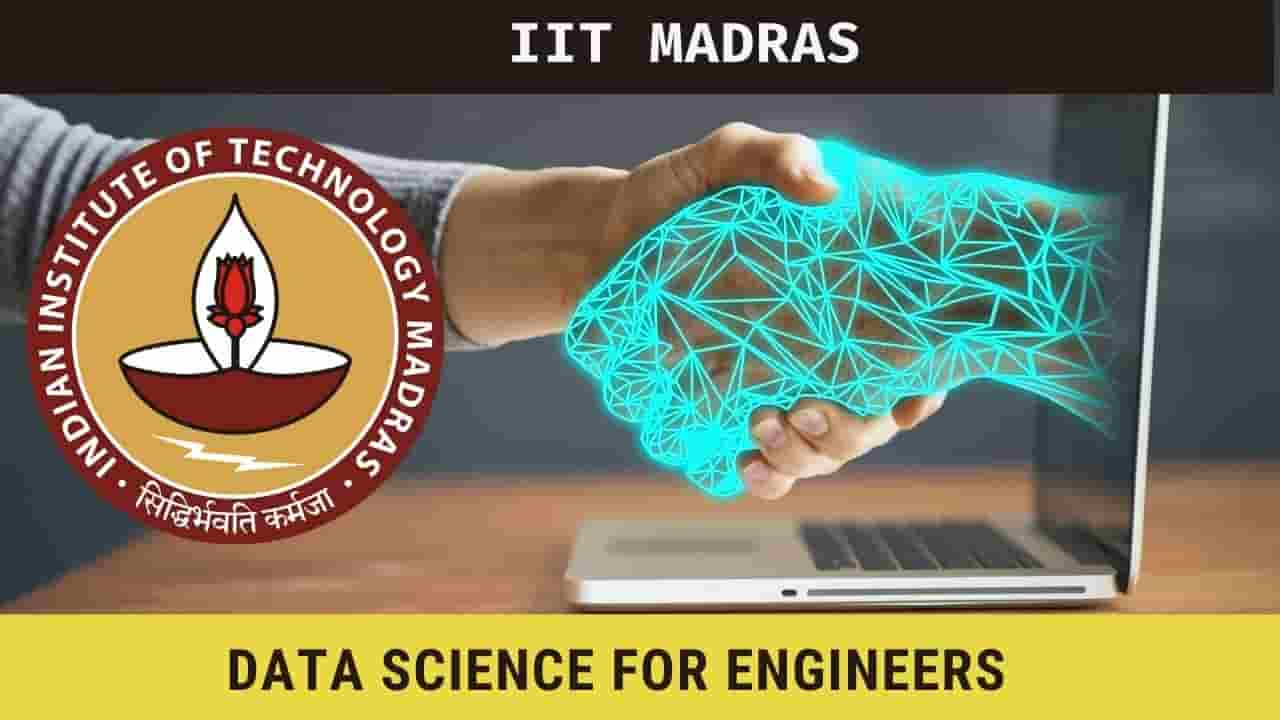Data Science for Engineers

## Overview

Indian Institute of Technology Madras, IIT Madras has launched the online course on Data Science for Engineers by the online platform Swayam. This course By Prof. Ragunathan Rengasamy, Prof. Shankar Narasimhan  . This is an 08-week online course starting from the 18 Jan 2021.

## Topics

The following topics will be covered in this online course,

• Course philosophy and introduction to R
• Linear algebra for data science
• Algebraic view - vectors, matrices, a product of matrix & vector, rank, null space, solution of an over-determined set of equations and pseudo-inverse)
• Geometric view - vectors, distance, projections, eigenvalue decomposition
• Statistics (descriptive statistics, the notion of probability, distributions, mean, variance, covariance, covariance matrix, understanding univariate and multivariate normal distributions, introduction to hypothesis testing, the confidence interval for estimates)
• Optimization
• Optimization
• Typology of data science problems and a solution framework
•  Simple linear regression and verifying assumptions used in linear regression
• Multivariate linear regression, model assessment, assessing the importance of different variables, subset selection
• Classification using logistic regression
• Classification using kNN and k-means clustering

## Eligibility

Any interested learner

## PREREQUISITES

10 hrs of pre-course material will be provided, learners need to practice this to be ready to take the course.

#### For more (source) IIT Madras FREE course on the Data Science for Engineers by SWAYAM

##### Create a FREE webpage for your event.